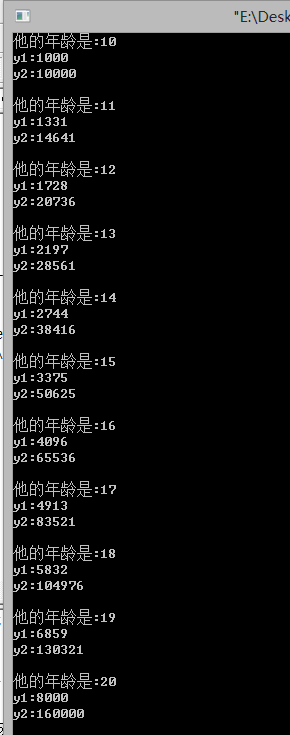# 猜年龄

美国数学家维纳(N.Wiener)智力早熟，11岁就上了大学。他曾在1935~1936年应邀来中国清华大学讲学。

一次，他参加某个重要会议，年轻的脸孔引人注目。于是有人询问他的年龄，他回答说：
“我年龄的立方是个4位数。我年龄的4次方是个6位数。这10个数字正好包含了从0到9这10个数字，每个都恰好出现1次。”

请你推算一下，他当时到底有多年轻。

#include<stdio.h>
#include<math.h>
#include<string.h>
int main()
{
int m,n,i,a,j;
for(i=10;i<30;i++)
{
m=pow(i,3); //pow（）函数的作用就是计算i的 3次方
n=pow(i,4);
memset(a,0,sizeof(a));//memset()函数在前的博客中已经提到 可以参见
if(m>=1000 && m<10000 && n>=100000 && n<1000000)//初步限定m为四位数 n为6位数
{
for(j=0;j<4;j++)
{
a[m%10]+=1; //因为在我们初始化的时候 a就是为了标记具体位置的 数字 用下表表示是不是存在这个数 标记为1
m/=10;
}
for(j=0;j<6;j++)
{
a[n%10]+=1;//同上 假如n为345678的话 也就是在下标为 3 4 5 6 7 8的位置是有数字的  也相当于存在了
n/=10;
}
for(j=0;j<10;j++)//因为总共有0 1 2 3 4 5 6 7 8 9 十个数
{
if (a[j]!=1) break;//此时的情况是 第j个数没有标记为1  即不符合要求
if (j==9) printf("%d\n",i);//就是遍历到最后一个未知的时候 也就是成立的情况
}
}
}
return 0;
}


#include<iostream>
#include<string.h>
using namespace std;

int year;
int y1,y2;
int main()
{
for(year=10;year<30;year++)
{
y1 = year*year*year;
y2 = year*year*year*year;
printf("他的年龄是:%d\n",year);
printf("y1:%d\n",y1);
printf("y2:%d\n\n",y2);
}
return 0;

}public class GuessYear {

/**
* 判断两个数组中的数字是否有相同的
* @param x
* @param y
* @return
*/
boolean isEqual(long[] x,long[] y){

boolean flag = false;
int len = x.length;
int len1 = y.length;

//判断两个数组间是否有相同的数字
for(int i = 0; i < len; i++){
for(int j = 0; j < len1; j++){
if(x[i] == y[j])
flag = true;
}
}
//判断第一个数组内是否有相同的数字
for(int i = 0; i < len; i++){
long tmp = x[i];
for(int j = i+1; j < len; j++){
if(tmp == x[j])
flag = true;
}
}
//判断第二个数组内是否有相同的数字
for(int i = 0; i < len1; i++){
long tmp = y[i];
for(int j = i+1; j < len1; j++){
if(tmp == y[j])
flag = true;
}
}

return flag;
}

/**
* 判断某数字是几位数
* @param tmp
* @return
*/
boolean isWhatBit(long num,int bit){
int count = 0;

while(num != 0){
num /= 10;
count++;
}

if( count == bit) return true;
else              return false;
}
/**
* 将数字分离到数组中
* @param a
* @param x
*/
void splitNumber(long num,long []x){
int i = x.length - 1;

while(num != 0){
x[i--] = num % 10;
num /= 10;
}
}
/**
* 猜年龄
*/
void guess(){

long x[] = new long;
long y[] = new long;

long row,col;

row = col = 10;
for(long i = 1; i < row; i++){
for(long j = 0; j < col; j++){
long tmp = i*10+j;

long f = tmp*tmp*tmp;
long s = f*tmp;

if(!isWhatBit(f,4))
continue;
if(!isWhatBit(s,6))
continue;

splitNumber(f,x);
splitNumber(s,y);

if(!isEqual(x,y))
System.out.println(tmp);

}
}
}
}  

12-1342002-17136
10-302768
11-14769
03-27499
02-0975
04-121181
03-061667
03-07183
01-05232
04-0530
01-053782
03-18180
01-25721
05-13485
06-211258
03-12240
03-18339
11-031135